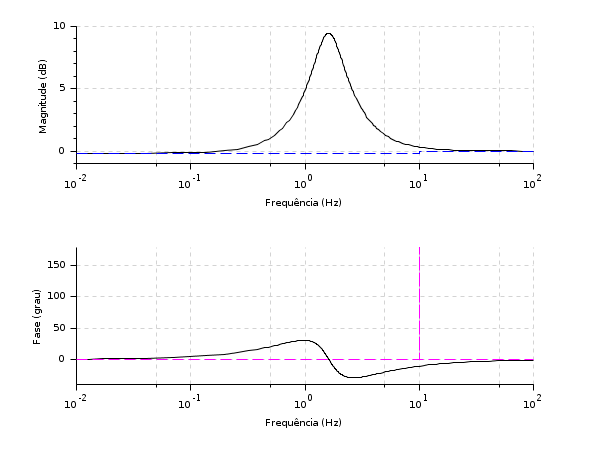Scilab Home page | Wiki | Bug tracker | Forge | Mailing list archives | ATOMS | File exchange
Change language to: English - Français - 日本語 - Русский

Ajuda do Scilab >> CACSD > Plot and display > bode_asymp

# bode_asymp

Bode plot asymptote

### Calling Sequence

bode_asymp(sl, [wmin, wmax])

### Arguments

sl

syslin list (SISO or SIMO linear system) in continuous time (type state-space or rational).

wmin, wmax

Real scalars: lower and upper frequency bounds (in rad/s).

### Description

Plots the asymptote of the system sl.

The optional arguments wmin and wmax (in rad/s) can be used to plot asymptote in a specific range of frequency.Note that the function bode() or gainplot() must be called before bode_asymp().Warning: this function is not applicable when the first input argument is a real matrix.

### Examples

s = poly(0, "s");
h = syslin("c", (s^2+2*0.9*10*s+100)/(s^2+2*0.3*10.1*s+102.01));

clf(); bode(h, 0.01, 100); bode_asymp(h);s = poly(0, "s");
h1 = syslin("c", (s^2+2*0.9*10*s+100)/(s^2+2*0.3*10.1*s+102.01));
h2 = syslin("c", (10*(s+3))/(s*(s+2)*(s^2+s+2)));

clf(); bode([h1; h2], 0.01, 100, ["h1"; "h2"]); bode_asymp([h1; h2]);s = %s;
G = (s+10)/(s*(s+100)); // A rational matrix
sys = syslin("c", G); // A continuous-time linear system in transfer matrix representation.
f_min = .0001; f_max = 1600; // Frequencies in Hz

bode_asymp(sys, 10, 1000); // Plots asymptotes in the given range of frequency (in rad/s).s = poly(0, "s");
h = syslin("c", (s^2+2*0.9*10*s+100)/(s^2+2*0.3*10.1*s+102.01));
h = tf2ss(h);

clf();
gainplot(h, 0.01, 100, "$\frac{s^2+18 s+100}{s^2+6.06 s+102.1}$"); // Frequencies in Hertz
bode_asymp(h)• bode — diagrama de Bode
• black — diagrama de Black (carta de Nichols)
• nyquist — diagrama de Nyquist
• gainplot — esboço de magnitude
• repfreq — frequency response
• g_margin — gain margin and associated crossover frequency
• p_margin — phase margin and associated crossover frequency
• calfrq — frequency response discretization
• phasemag — phase and magnitude computation
• datatips — Tool for placing and editing tips along the plotted curves.
• show_margins — display gain and phase margin and associated crossover frequencies

### History

 Versão Descrição 5.5.0 bode_asymp() function introduced.